# Answered Essay: Applying Kirchoff's current law at each of the nodes (black dots), and using the current directions indicated b

matlab language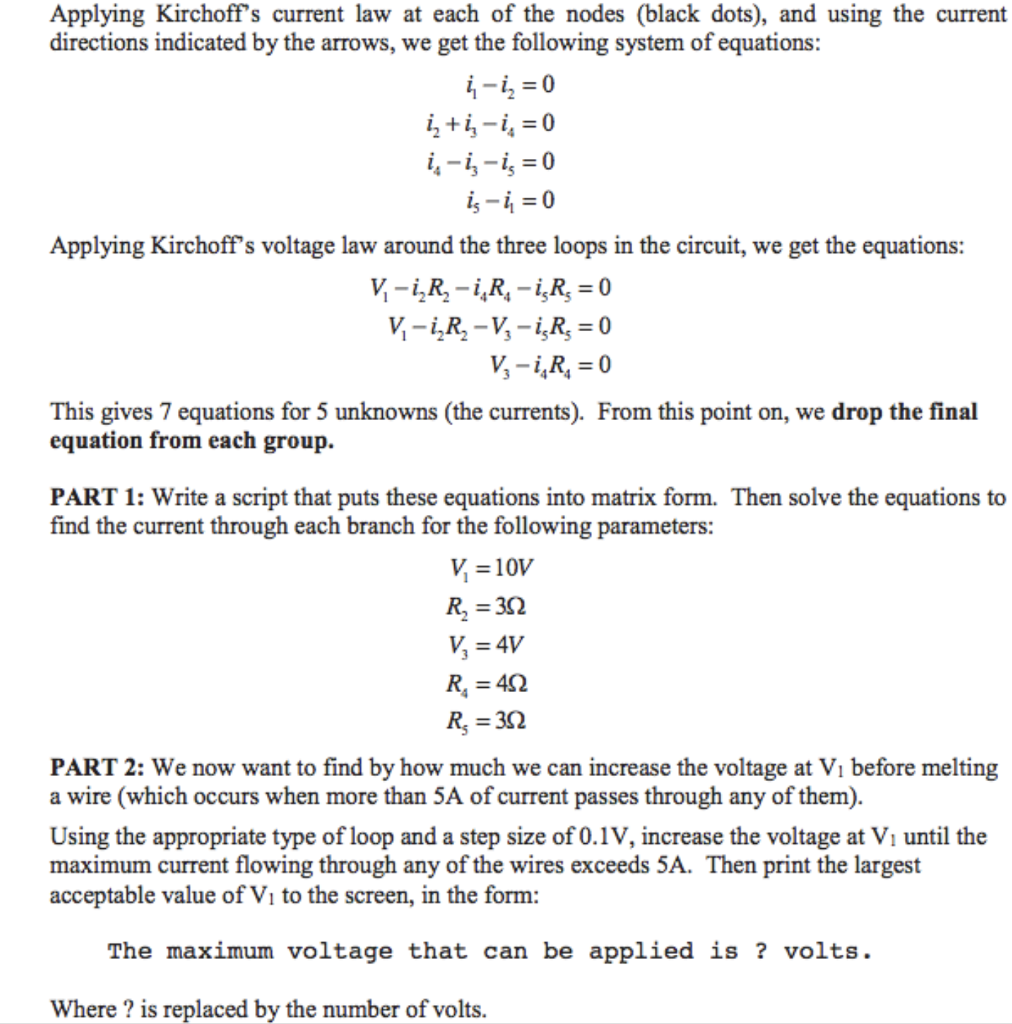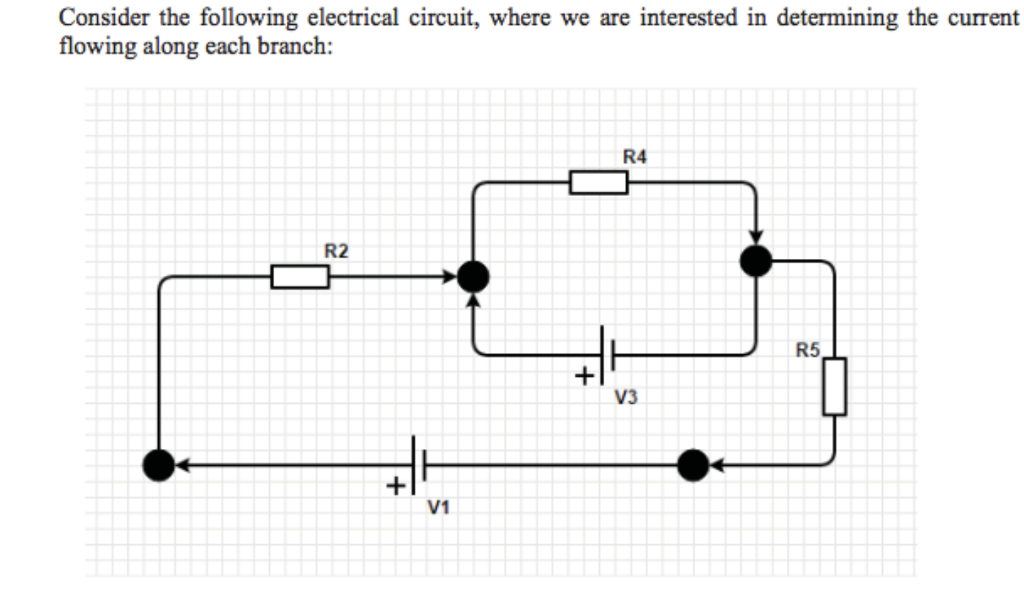Applying Kirchoff’s current law at each of the nodes (black dots), and using the current directions indicated by the arrows, we get the following system of equations: i_1 – i_2 =0 i_2 + i_3 – i_4 i_4 – i_3 – i_5 = 0 i_5 – i_1 = 0 Applying Kirchoff’s voltage law around the three loops in the circuit, we get the equations: V – i_2R_2 – i_4R_4 – i_5R_5 = 0 V_1 – i_2R_2 – V_3 – i_5R_5 = 0 V_3 – i_4R_4 = 0 This gives 7 equations for 5 unknowns (the currents). From this point on, we drop the final equation from each group. Write a script that puts these equations into matrix form. Then solve the equations to find the current through each branch for the following parameters: V_1 =10V R_2 = 3 Ohm V_3 = 4V R_4 = 4 Ohm R_5 = 3 Ohm We now want to find by how much we can increase the voltage at V_1 before melting a wire (which occurs when more than 5A of current passes through any of them). Using the appropriate type of loop and a step size of 0.1V, increase the voltage at V_1 until the maximum current flowing through any of the wires exceeds 5A. Then print the largest acceptable value of V_1 to the screen, in the form: The maximum voltage that can be applied is ? volts. Where? is replaced by the number of volts.

Part 1:

​The matrix is formed to solve the system of linear equation of the form AX=B where X is a 5-by-1 vector containing current values i1,i2,i3,i4 and i5. Below is the matlab code with comments describing matrices and after that output is attached.

Matlab code:

% ###################### 1 #########################
% variable to calculate are currents i1, i2, i3, i4, i5
syms i1 i2 i3 i4 i5
% given values
v1 = 10;
r2 = 3;
v3 = 4;
r4 = 4;
r5 = 3;

% given 5 equations
eqn1 = i1-i2 == 0;
eqn2 = i2+i3-i4 == 0;
eqn3 = i4-i3-i5 == 0;
eqn4 = v1-i2*r2-i4*r4-i5*r5 == 0;
eqn5 = v1-i2*r2-v3-i5*r5 == 0;

% equation matrix AX = B
[A,B] = equationsToMatrix([eqn1, eqn2, eqn3, eqn4, eqn5], [i1, i2, i3, i4, i5]);

% solving equation AX = B, X is a vector containing 5 current values
X = linsolve(A,B)
A
B

Output part 1:

matrix A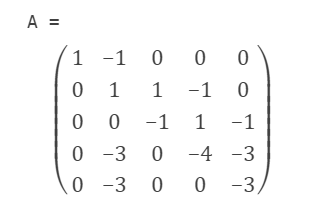matrix B: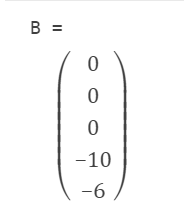​matrix X( solution containing currents i1, i2, i3, i4, i5):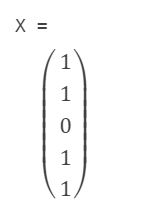​Part 2:

​In part 2, voltage V1 is set as an variable and matrix is solved similarily as in part 1 but V1 as an variable. Which produces solution in terms of variable V1 which is found to be: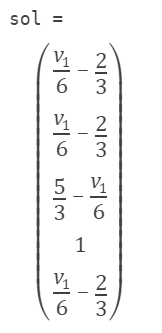Putting V1 = 10 in the above solution gives answer same as in part 1. Now initialize V1= 10 V and calculate currents by putting V1 = 10 in above solution. Now in a while loop increase V1 by 0.1 in each step , calculate new currents by putting this V1 value in above solution matrix until either of i1, i2, i3, i4 or i5 gets 5A. Finally print V1.

Matlab Code:

%##################### 2 #######################
% now put v1 also an variable
syms i1 i2 i3 i4 i5 v1
% rest constants as it is
r2 = 3;
v3 = 4;
r4 = 4;
r5 = 3;

% define equations
eqn1 = i1-i2 == 0;
eqn2 = i2+i3-i4 == 0;
eqn3 = i4-i3-i5 == 0;
eqn4 = v1-i2*r2-i4*r4-i5*r5 == 0;
eqn5 = v1-i2*r2-v3-i5*r5 == 0;

% equation matrix
[A,B] = equationsToMatrix([eqn1, eqn2, eqn3, eqn4, eqn5], [i1, i2, i3, i4, i5]);

% solve system in terms of v1
sol = linsolve(A,B)

% initial value of v1
v1_init = 10;
% put v1= 10 in system of equation solution
currents = subs(sol,v1,v1_init);
% while currents less than 5
while double(currents(1))<5 && double(currents(2))<5 && double(currents(3))<5 && double(currents(4))<5 && double(currents(5))<5
v1_init = v1_init+0.1;
currents = subs(sol,v1,v1_init);
end

% print final value of v1 when any current gets 5
fprintf(‘The maximum volatge that can be applied is %2.2f volts.’,v1_init);
% print currents at that time
currents

Output part 2: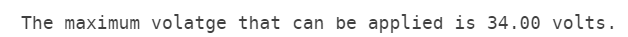Currents at this voltage: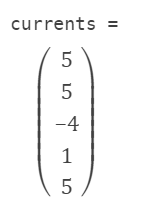Calculate your paper price
Pages (550 words)
Approximate price: -

Help Me Write My Essay - Reasons:Best Online Essay Writing Service

We strive to give our customers the best online essay writing experience. We Make sure essays are submitted on time and all the instructions are followed.Our Writers are Experienced and Professional

Our essay writing service is founded on professional writers who are on stand by to help you any time.Free Revision Fo all Essays

Sometimes you may require our writers to add on a point to make your essay as customised as possible, we will give you unlimited times to do this. And we will do it for free.Timely Essay(s)

We understand the frustrations that comes with late essays and our writers are extra careful to not violate this term. Our support team is always engauging our writers to help you have your essay ahead of time.Customised Essays &100% Confidential

Our Online writing Service has zero torelance for plagiarised papers. We have plagiarism checking tool that generate plagiarism reports just to make sure you are satisfied.Our agents are ready to help you around the clock. Please feel free to reach out and enquire about anything.

Try it now!

## Calculate the price of your order

Total price:
\$0.00

How it works?

Follow these simple steps to get your paper doneFill in the order form and provide all details of your assignment.Proceed with the payment

Choose the payment system that suits you most.Receive the final file

Once your paper is ready, we will email it to you.

HOW OUR ONLINE ESSAY WRITING SERVICE WORKS

Let us write that nagging essay.## Submit Your Essay/Homework Instructions

By clicking on the "PLACE ORDER" button, tell us your requires. Be precise for an accurate customised essay. You may also upload any reading materials where applicable.Pick A & Writer

Our ordering form will provide you with a list of writers and their feedbacks. At step 2, its time select a writer. Our online agents are on stand by to help you just in case.Editing (OUR PART)

At this stage, our editor will go through your essay and make sure your writer did meet all the instructions.